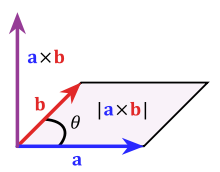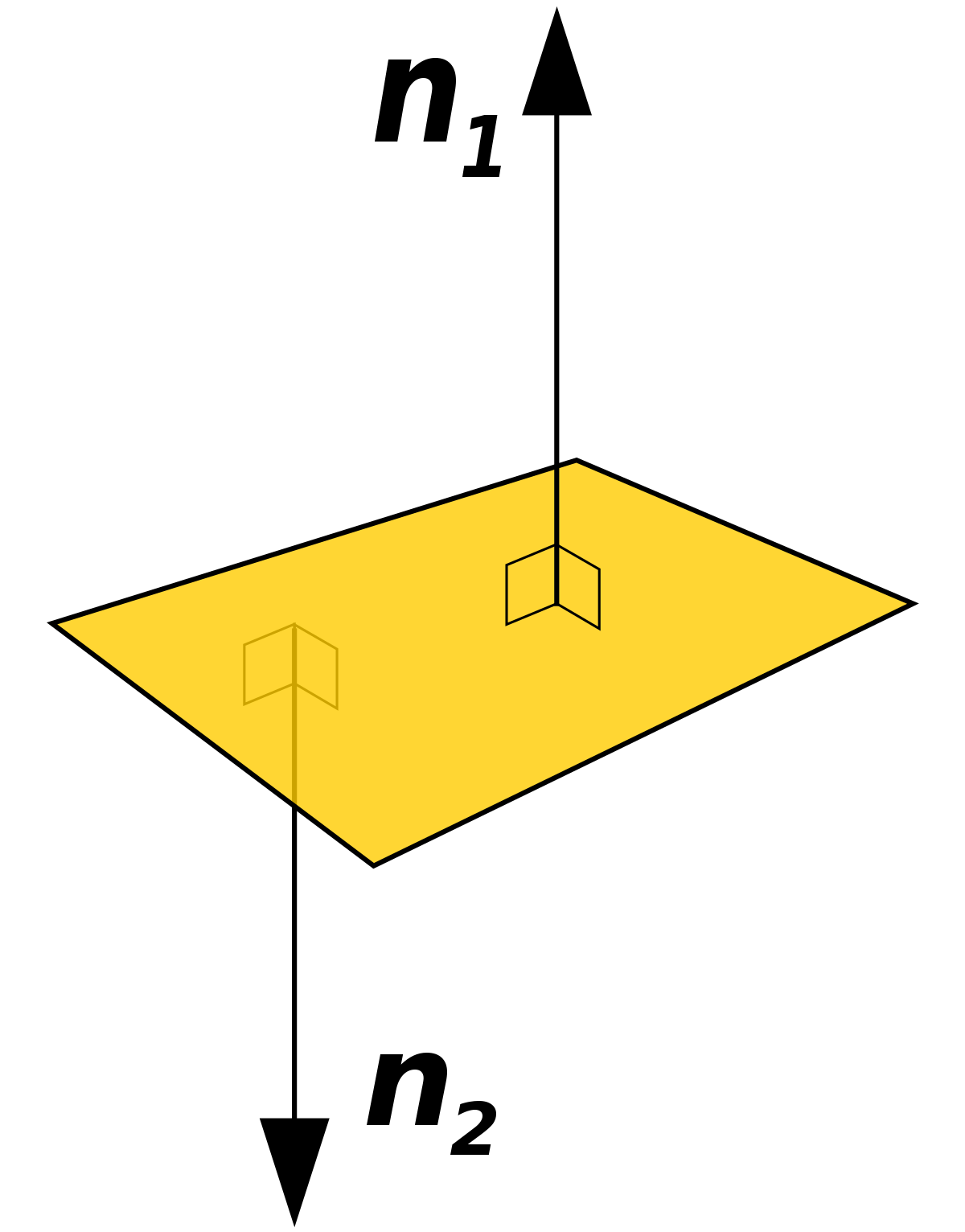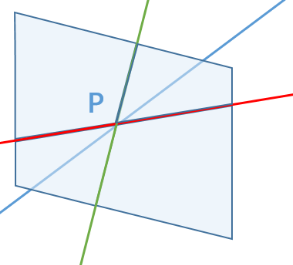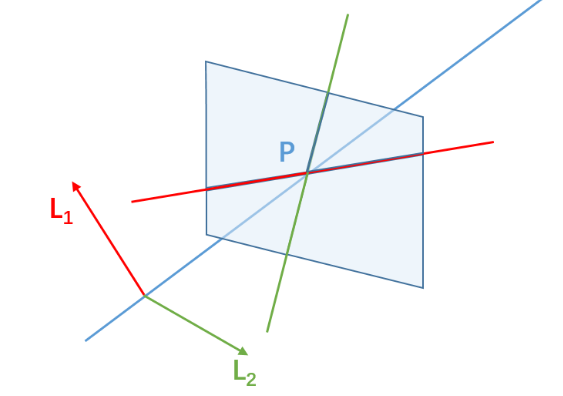# Recap

In Part 1 we saw how reframing concepts in math can lead to new and interesting ways of seeing the world. In Part 2 we applied the reframing idea to familiar ideas from geometry and found a deep connection between lines and planes and vectors. But why go to all the trouble of rearranging everything we learned in geometry? Why does this matter?

# Mathematical background

In order to fully appreciate what we’re about to see, we will need to begin with some mathematical background. If you know what equivalence classes and cross-products are, you know enough to skip this section.

Geometry: points, lines, rays, planes, spaces

We'll be using the basic language of geometry to describe how images appear on flat surfaces. The internet is filled with introduction to geometry tutorials if you want to brush up. Don't worry too much about fancy theorems, just understand what points, lines, rays, planes and spaces are.

Vectors: direction, magnitude, cross-products. Normal vectors for planes

Vectors have direction and size. Whereas regular numbers can be big or small, vectors also must go in a certain direction. If you've ever given directions to someone, you instinctively understand this. You don't say "walk four city blocks," you say "walk four city blocks towards the east." Otherwise they wouldn't know which way to go.

There are mathematical operations on vectors the same way we have mathematical operations on numbers (like addition, multiplication, and so on). One such operation is called the cross-product, which takes two vectors and produces a third vector that is perpendicular to the other two.Imagine vectors a and b are lying flat on a table. Then a x b is the cross-product which is pointing straight up. The main thing you need to know about cross-products is that the resulting vector is at a right angle to the other two.

If you have a flat surface (a plane), you can find vectors that are perpendicular to the entire plane. Such vectors are also called normal vectors.One way to find these normal vectors is by taking the cross-product of two vectors in the plane. Since the two vectors are both in the plane, the cross-product will be perpendicular to both of them, and therefore perpendicular to the plane as a whole.

# Why it matters

To see why this new approach is so powerful, we have to ask a natural follow-up question: if we have two lines in the projective plane, how do we find where they intersect?What do we know about point P?

Recall: each line has a normal vector (L1, L2). It turns out that if we take the cross-product of these two lines and you get a vector that lies on the blue line and points you straight to the intersection P.That’s it!

That’s the entire process for finding where two lines meet.

The real beauty of our new way of framing geometry is that it lets us do things like this in a very simple manner. That’s the real reason we’ve spent so much time building out this crazy frame. Being able to simply say P = L1 x L2 is a lot easier than writing a whole system of equations and trying to solve them, isn’t it?

Before you keep going, take a quick second to breathe. Get up, go get something to drink, use the bathroom, whatever. Because Part 4 is going to be an express ticket, no-stops-in-between, all-out ride to a sparkling conclusion.